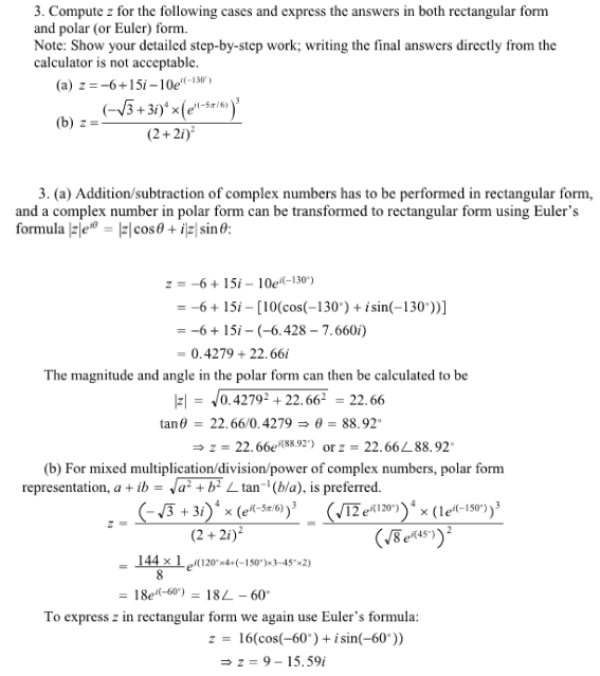Compute z for the following cases and express the answers in both rectangular form and polar (or Euler) form. Note: Show your detailed step-by-step work; writing the final answers directly from the calculator is not acceptable. (a) z=-6+15i-10e^i-130 degrees (b) z=((-3^1/2+3i)^4(e^i(-5pi/6)))/(2+2i)^2 .Compute z for the following cases and express the answers in both rectangular form and polar (or Euler) form. Note: Show your detailed step-by-step work; writing the final answers directly from the calculator is not acceptable. (a) z=-6+15i-10e^i-130 degrees (b) z=((-3^1/2+3i)^4(e^i(-5pi/6)))/(2+2i)^2 .

System Dynamics Page 2 dynamics dynamics dynamics dynamics dynamics dynamics dynamics System dynamics Page 3